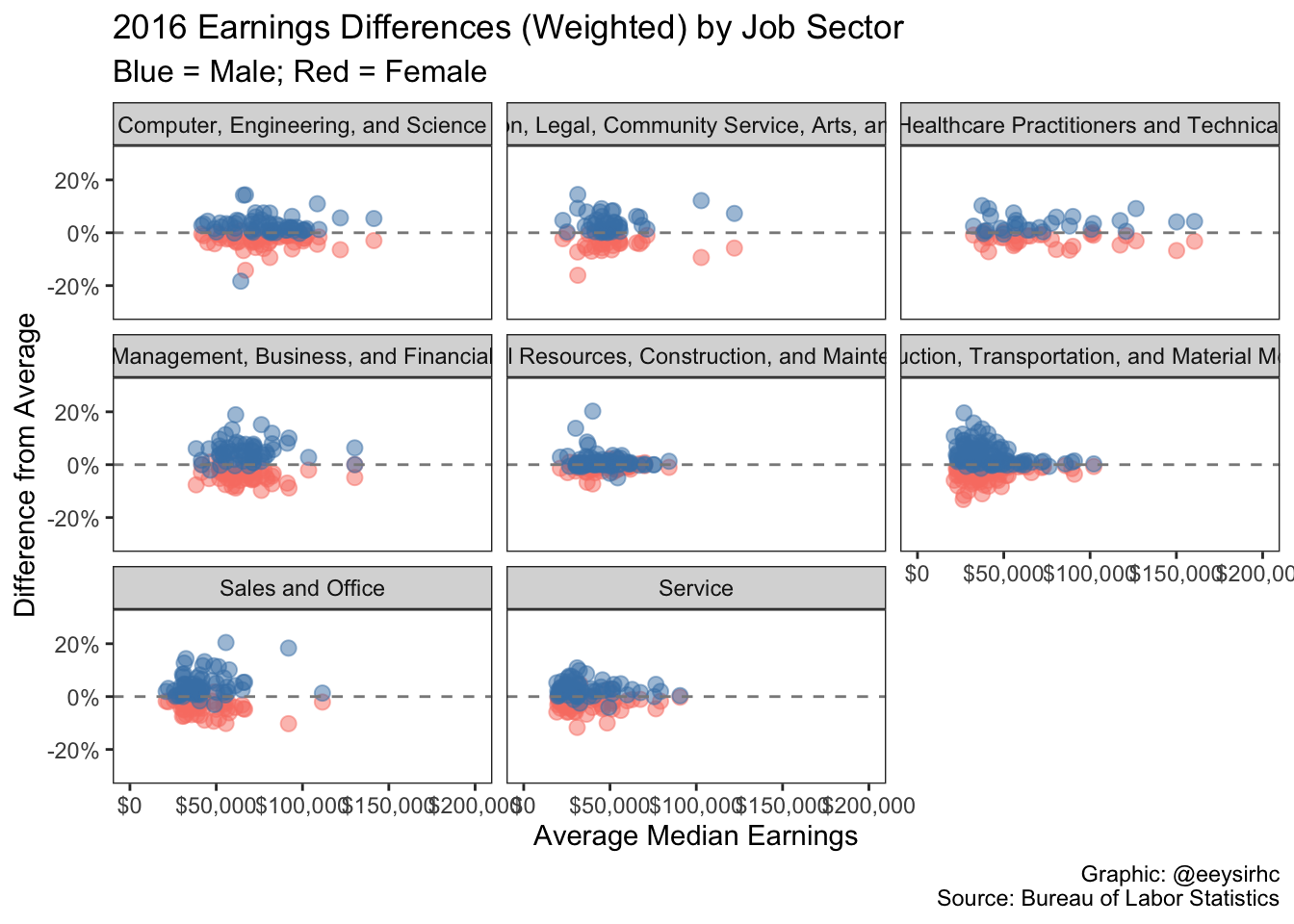Analyzing data for #tidytuesday week of 3/05/2019 (source)

``````library(tidyverse)
library(scales)
library(lubridate)

## Clean & plot data

``````jobs_gender %>%
filter(year == '2016') %>%
mutate(male_diff = ((((total_earnings_male/total_earnings)-1)*workers_male)/total_workers),
female_diff = (((total_earnings_female/total_earnings)-1)*workers_female)/total_workers) %>%
ggplot() +
geom_jitter(aes(total_earnings, female_diff),
color = 'salmon',
alpha = 0.5,
size = 2.5) +
geom_jitter(aes(total_earnings, male_diff),
color = 'steelblue',
alpha = 0.5,
size = 2.5) +
geom_hline(yintercept = 0, color = 'grey54', lty = 'dashed') +
facet_wrap(~major_category) +
scale_x_continuous(labels = dollar_format(),
limits = c(0,200000)) +
scale_y_continuous(labels = percent_format(round(1)),
limits = c(-0.3,0.3)) +
labs(x = "Average Median Earnings",
y = "Difference from Average",
caption = "Graphic: @eeysirhc\nSource: Bureau of Labor Statistics",
title = "2016 Earnings Differences (Weighted) by Job Sector",
subtitle = "Blue = Male; Red = Female") +
theme_bw() +
theme(panel.grid.major = element_blank(),
panel.grid.minor = element_blank(),
plot.subtitle = element_text(size = 12),
legend.position = 'none')``````